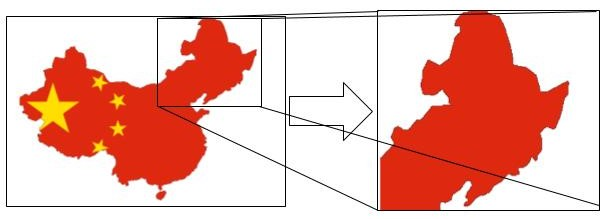19 / 32

# Numpy - Arrays - Example - Extracting a portion of an image using array slicing

Let us extract a portion of an image using NumPy array slicing.

We can represent an image by arranging its pixel values in the form of a NumPy array.

Then, by slicing this NumPy array in desired dimensions of the pixel locations of the image, we can extract the desired portion of this image.

e.g.

we can extract a portion of `china` image by specifying the pixel locations (in NumPy array) of this image portion.

Let us say dimensions of `china` image (`china.shape`) is 427 x 640 x 3. And we want to extract the portion of this image, and dimensions of this portion are - row numbers from 150 to 220 and column numbers from 130 to 250 in the 'china' NumPy array.

To extract this portion, we can simply use the below code statement

``````image = china[150:220, 130:250]
``````

Here, `china` is the NumPy array representing the image of china, and `image` is the desired portion of this image, that we wanted to extract.INSTRUCTIONS

(1) Please import `load_sample_image` from `sklearn.datasets`

``````china = load_sample_image("<<your code comes here>>")
``````

(3) Please extract a portion of `china` image by extracting row numbers from 120 to 250 and column numbers from 110 to 230, and store this image portion in a variable called 'portion'

``````portion = china[<<your code comes here>>]
``````

(4) Check the dimensions of the `portion` image by printing the shape of the `portion` array

``````print(<<your code comes here>>)
``````

No hints are availble for this assesment

Note - Having trouble with the assessment engine? Follow the steps listed here